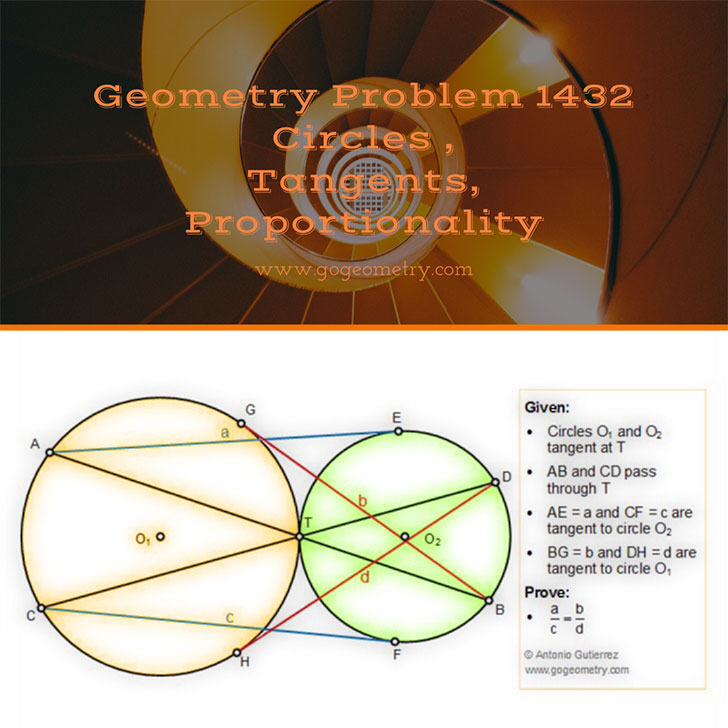# Geometry Problem 1432: Tangent Circles, Secant, Tangent Lines, Proportionality, Similarity

The figure below shows circle O1 and O2 tangent at T. Lines AB and CD pass through T. AE = a and CF = c are tangent to circle O2. BG = b and DH = d are tangent to circle O1. Prove that $${a \over c} = {b \over d}$$.## Typography and Sketching of Problem 1432 using iPad Apps